# Batman vs. The Penguin Debate

Due Thursday! Watch “Batman vs. The Penguin Debate.” (Link follows, or check MyD.)

Batman vs. The Penguin Debate

Answer these five questions in a new entry on your WordPress blog (call it “Batman vs. The Penguin Debate”):

1. What is The Penguin’s main idea? What are his reasons?

2. What evidence does he use to support his reasons?

3. How does Batman start his rebuttal? (When two people debate, one of them makes an argument, followed by a rebuttal from the other person. The rebuttal basically states, “No, you’re wrong, and this is why.”)

4. What kind of tactics to you think are fair or unfair in debating, based on this example?

5. Who seems to have the best manner? Why?

# Geometry Standard Review Guide

Geometry Standard:

Topics include: perimeter, area and volume. Need to know the formulas for basic shapes of rectangles and triangles. For trapezoids and other shapes, the formulas will be provided.

Math Notes:

Practice Problems CL 1-99CL 2-985-805-915-92, and 5-101. Problems 9-109-119-249-259-369-37, and 9-61

Area practice problems and answers found in this Unit 5 Parent Guide

Volume and Surface Area practice problems and answers found in the Unit 9 Parent Guide

# Ratios and Proportions Standard Review Guide

Ratios and Proportions:

Topics include: unit rates, ratios, proportions

Math Notes:

Math Problems: 3-333-844-805-616-90CL 7-132,  7-87-9(c),  7-10(c)7-147-19,  7-30 ,7-247-267-30, and 7-688-90, Problem 8-101,
Many problems and answers to practice with on this Parent Guide for Unit 7

# Number System Standard Review Guide

Number System:

Topics include: Multiplying and dividing fractions and decimals. Division methods: common denominators, Super Giant One, Multiplicative Inverse.

Checkpoint 8B

Math Notes:

Math Problems: CL 4‑905‑5,5‑27, and 5‑35, Problems CL 6-1247-527-647-74, and 7-99(a), Problems CL 6-1247-55, and 7-75

# Expressions and Equations Standard Review Guide

Expressions and Equations Standard

Topics include: writing expressions, distributive property, combining like terms, evaluating (substitute and solve!) expressions and equations.

Checkpoint 8A

Math Notes:

Math Problems 6-866-966-106, and 6-115 , Problems CL 4-86,6-75, and 6-110, Problem 6-107, Problems 6-72 and6-98,Problems 7-937-957-1037-111, and 7-121, Problems CL 4-875-165-515-104CL 6-121, andCL 6-122

# Data And Statistics Standards Review Guide

Monday, May 26th: Data and Statistics Standard

Topics include: Histograms, Stem and Leaf Plots, Box and Whisker Plots, CEntral Tendencies, Mean vs. Median.

Math Notes:

Chapter 8 Problems 8-208-218-398-408-51, and 8-91# Checkpoint 9A

Problem 9-39
Displays of Data: Histograms and Box Plots
 Answers to problem 9-39: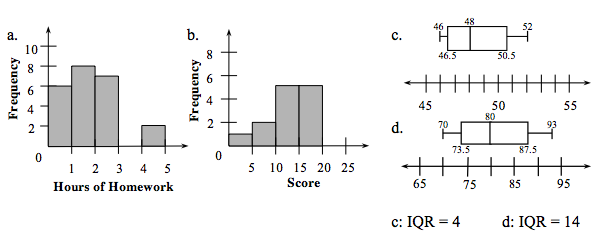HistogramsA histogram is a method of showing data. It uses a bar to show the frequency (the number of times something occurs). The frequency measures something that changes numerically. (In a bar graph the frequency measures something that changes by category.) The intervals (called bins) for the data are shown on the horizontal axis and the frequency is represented by the height of a rectangle above the interval. The labels on the horizontal axis represent the lower end of each interval or bin. Example: Sam and her friends weighed themselves and here is their weight in pounds: 110, 120, 131, 112, 125, 135, 118, 127, 135, and 125. Make a histogram to display the information. Use intervals of 10 pounds.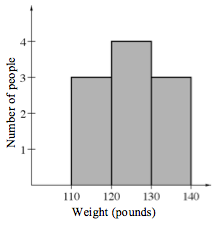Solution: See histogram at right. Note that the person weighing 120 pounds is counted in the next higher bin. Box PlotsA box plot displays a summary of data using the median, quartiles, and extremes of the data. The box contains the “middle half” of the data. The right segment represents the top 25% of the data and the left segment represent the bottom 25% of the data. Example: Create a box plot for the set of data given in the previous example. S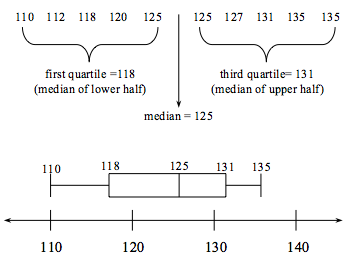olution:Place the data in order to find the median (middle number) and the quartiles (middle numbers of the upper half and the lower half.) Based on the extremes, first quartile, third quartile, and median, the box plot is drawn. The interquartile range IQR = 131–118 = 13. Now we can go back to the original problem. The 0–1 bin contains the six students who do less than one hour of homework. The 1–2 bin contains the 10 students who do at least one hour but less than two hours. The 2–3 bin contains the seven students who do at least two hours but less than three hours. There are no students who do at least three hours and less than four. Two students did four hours and less than five. See the histogram above. The 0–5 bin contains two scores less than 5 points. The 5–10 bin contains the two scores of a least five but less than 10. The 10–15 bin contains the eight scores at least 10 but less than 15. The 15–20 bin contains the seven scores at least 15 but less than 20. See the histogram above. Place the ages in order: 46, 46, 47, 47, 48, 49, 50, 51, 52. The median is the middle age: 48. The first quartile is the median of the lower half of the ages. Since there are four lower-half ages, the median is the average of the middle two: . The third quartile is the median of the upper half ages. Again, there are four upper-half ages, so average the two middle ages: . The interquartile range is the difference between the third quartile and the first quartile: 50.5–46.5 = 4. See the box plot above. Place the scores in order: 70, 72, 75, 76, 80, 82, 85, 90, 93. The median is the middle score: 80. The lower quartile is the median of the lower half of the scores. Since there are four lower-half scores, the median is the average of the middle two: . The third quartile is the median of the upper half of the scores. Again, there are four upper-half scores, so average the two middle ages: . The interquartile range is the difference between the third quartile and the first quartile: 87.5–73.5 = 14. See the box plot above. Here are some more to try. For problems 1 through 6, create a histogram. For problems 7 through 12, create a box plot. State the quartiles and the interquartile range. 1. Number of heads showing in 20 tosses of three coins: 2, 2, 1, 3, 1, 0, 2, 1, 2, 1, 1, 2, 0, 1, 3, 2, 1, 3, 1, 2 2. Number of even numbers in 5 rolls of a dice done 14 times: 4, 2, 2, 3, 1, 2, 1, 1, 3, 3, 2, 2, 4, 5 3. Number of fish caught by 7 fishermen: 2, 3, 0, 3, 3, 1, 5 4. Number of girls in grades K-8 at local schools: 12, 13, 15, 10, 11, 12, 15, 11, 12 5. Number of birthdays in each March in various 2nd grade classes: 5, 1, 0, 0, 2, 4, 4, 1, 3, 1, 0, 4 6. Laps jogged by 15 students: 10, 15, 10, 13, 20, 14, 17, 10, 15, 20, 8, 7, 13, 15, 12 7. Number of days of rain: 6, 8, 10, 9, 7, 7, 11, 12, 6, 12, 14, 10 8. Number of times a frog croaked per minute: 38, 23, 40, 12, 35, 27, 51, 26, 24, 14, 38, 41, 23, 17 9. Speed in mph of 15 different cars: 30, 35, 40, 23, 33, 32, 28, 37, 30, 31, 29, 33, 39, 22, 30 10. Typing speed of 12 students in words per minute: 28, 30, 60, 26, 47, 53, 39, 42, 48, 27, 23, 86 11. Number of face cards pulled when 13 cards are drawn 15 times: 1, 4, 2, 1, 1, 0, 0, 2, 1, 3, 3, 0, 0, 2, 1 12. Height of 15 students in inches: 48, 55, 56, 65, 67, 60, 60, 57, 50, 59, 62, 65, 58, 70, 68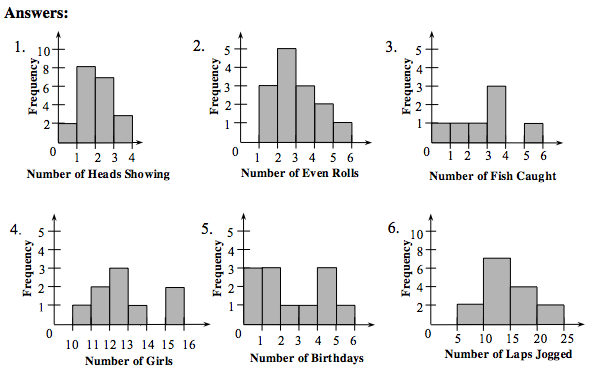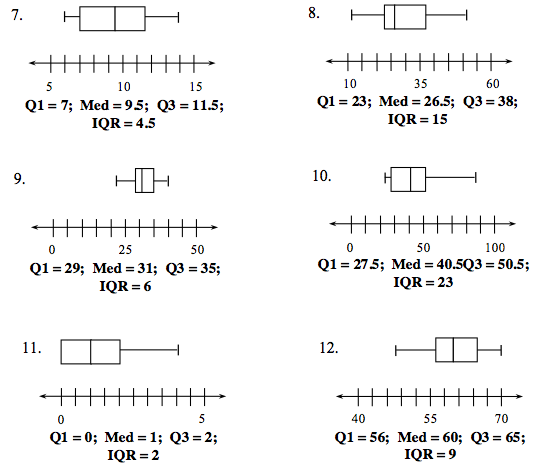# Land vs. Sea Breezes